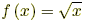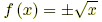index: click on a letter A B C D E F G H I J K L M N O P Q R S T U V W X Y Z A to Z index index: subject areas numbers & symbols sets, logic, proofs geometry algebra trigonometry advanced algebra & pre-calculus calculus advanced topics probability & statistics real world applications multimedia entrieswww.mathwords.com about mathwords website feedback

 Function A relation for which each element of the domain corresponds to exactly one element of the range. For example,is a function because each number x in the domain has only one possible square root. On the other hand,is not a function because there are two possible values for any positive value of x.     See also Vertical line test